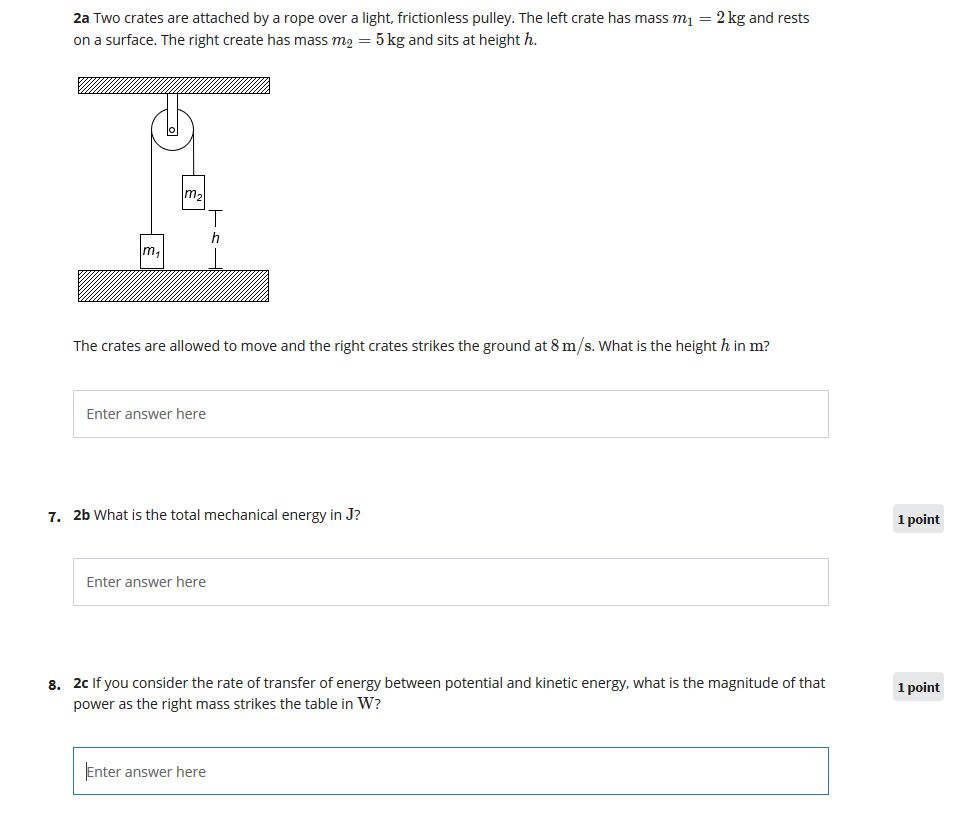# Question Solved1 AnswerTwo crates are attached by a rope over a light, frictionlesspulley. The left crate has mass 2kg and rests on a surface, theright crate has mass 5kg and sits at a height h.Note: I have solved (a) and (b). I cannot make2c work. 392 W is wrong, 196.5W is wrong (found ina previous question asked on this site), 84W is wrong. No matterwhich energy I choose it's wrong. How do I solve this? 2a Two crates are attached by a rope over a light, frictionless pulley. The left crate has mass m1 = 2 kg and rests on a surface. The right create has mass m2 = 5 kg and sits at height h. m2 h m The crates are allowed to move and the right crates strikes the ground at 8 m/s. What is the height h in m? Enter answer here 7. 2b What is the total mechanical energy in J? 1 1 point Enter answer here 8. 2c If you consider the rate of transfer of energy between potential and kinetic energy, what is the magnitude of that power as the right mass strikes the table in W? 1 point Enter answer hereTwo crates are attached by a rope over a light, frictionless pulley. The left crate has mass 2kg and rests on a surface, the right crate has mass 5kg and sits at a height h.Note: I have solved (a) and (b). I cannot make 2c work. 392 W is wrong, 196.5W is wrong (found in a previous question asked on this site), 84W is wrong. No matter which energy I choose it's wrong. How do I solve this?

Transcribed Image Text: 2a Two crates are attached by a rope over a light, frictionless pulley. The left crate has mass m1 = 2 kg and rests on a surface. The right create has mass m2 = 5 kg and sits at height h. m2 h m The crates are allowed to move and the right crates strikes the ground at 8 m/s. What is the height h in m? Enter answer here 7. 2b What is the total mechanical energy in J? 1 1 point Enter answer here 8. 2c If you consider the rate of transfer of energy between potential and kinetic energy, what is the magnitude of that power as the right mass strikes the table in W? 1 point Enter answer here
More
Transcribed Image Text: 2a Two crates are attached by a rope over a light, frictionless pulley. The left crate has mass m1 = 2 kg and rests on a surface. The right create has mass m2 = 5 kg and sits at height h. m2 h m The crates are allowed to move and the right crates strikes the ground at 8 m/s. What is the height h in m? Enter answer here 7. 2b What is the total mechanical energy in J? 1 1 point Enter answer here 8. 2c If you consider the rate of transfer of energy between potential and kinetic energy, what is the magnitude of that power as the right mass strikes the table in W? 1 point Enter answer here